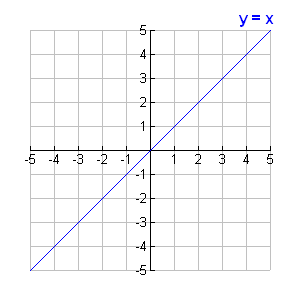# Plotting a graph in four quadrant

Hello,

``````     I want to plot a graph of let's say y = x by reading data from a data file in four quadrants in ROOT. How can i do it?
``````

can anybody give me some information?

I guess you can divide a TCanvas in 4 parts and draw a graph in each … Do you have an example showing the kind of plot you want ?

Hello sir,
Thank you for your kind reply. I am attaching a plot that i want to plot using root . What i have done is basically, I made a for loop to generate data points of y = x by varying x from -300 to +300. By reading the data points from the data file , i plotted a graph. I am attaching two plots of y = x below. One is obtained from root and other i got it by searching over google. What i want is to plot it in four quadrants .graph.pdf (38.3 KB)

here is an example:

``````{
c = new TCanvas("c","XY plot",200,10,700,500);
c->SetFillColor(0);
c->SetFrameLineColor(10);
c->SetFrameBorderMode(0);

const Int_t n = 4;
Double_t x[n] = {-1, -3, -9, 3};
Double_t y[n] = {-1000,  900,  300, 300};
gr = new TGraph(n,x,y);
gr->SetTitle("XY plot");
gr->SetMinimum(-1080);
gr->SetMaximum(1080);
gr->Draw("AC*");

gr->GetHistogram()->GetYaxis()->SetTickLength(0);
gr->GetHistogram()->GetXaxis()->SetTickLength(0);
gr->GetHistogram()->GetYaxis()->SetLabelOffset(999);
gr->GetHistogram()->GetXaxis()->SetLabelOffset(999);
gr->GetHistogram()->GetXaxis()->SetAxisColor(0);
gr->GetHistogram()->GetYaxis()->SetAxisColor(0);

TGaxis *yaxis = new TGaxis(0, gPad->GetUymin(),
yaxis->Draw();
TGaxis *xaxis = new TGaxis(gPad->GetUxmin(), 0,
xaxis->Draw();
}``````

Hello sir,
Thank you so much. This code worked as well. I am trying to understand the whole code. I need to understand this TGaxis given below. How can i set the X and Y axis title ?

TGaxis *yaxis = new TGaxis(0, gPad->GetUymin(),
yaxis->Draw();
TGaxis *xaxis = new TGaxis(gPad->GetUxmin(), 0,

``````void xyplot ()
{
TCanvas *c = new TCanvas("c","XY plot",200,10,700,500);

// Remove the frame
c->SetFillColor(kWhite);
c->SetFrameLineColor(kWhite);
c->SetFrameBorderMode(0);

const Int_t n = 4;
Double_t x[n] = {-1, -3, -9, 3};
Double_t y[n] = {-1000,  900,  300, 300};
gr = new TGraph(n,x,y);
gr->SetTitle("XY plot");
gr->SetMinimum(-1080);
gr->SetMaximum(1080);
gr->SetLineColor(kRed);
gr->Draw("AC*");

// Remove the frame's axis
gr->GetHistogram()->GetYaxis()->SetTickLength(0);
gr->GetHistogram()->GetXaxis()->SetTickLength(0);
gr->GetHistogram()->GetYaxis()->SetLabelSize(0);
gr->GetHistogram()->GetXaxis()->SetLabelSize(0);
gr->GetHistogram()->GetXaxis()->SetAxisColor(0);
gr->GetHistogram()->GetYaxis()->SetAxisColor(0);

// Draw orthogonal axis system entered at (0,0)
TGaxis *yaxis = new TGaxis(0, gPad->GetUymin(),
yaxis->Draw();
TLatex *ytitle = new TLatex(-0.5,gPad->GetUymax(),"Y axis");
ytitle->Draw();
ytitle->SetTextSize(0.03);
ytitle->SetTextAngle(90.);
ytitle->SetTextAlign(31);
TGaxis *xaxis = new TGaxis(gPad->GetUxmin(), 0,
xaxis->Draw();
TLatex *xtitle = new TLatex(gPad->GetUxmax(),-200.,"X axis");
xtitle->Draw();
xtitle->SetTextAlign(31);
xtitle->SetTextSize(0.03);
}
``````

Hello sir,
Thank you so much . It worked as well. I am very happy. Actually I am learning root. I have to know so many things. I am trying my best. This is a good disscussion forum of root problems. I am very happy that, I have been included in the group .

With regards,
Prasant

You are welcome !Hello sir,
Can you please help me, how can i add GRID to the canvas. what i am thinking is to create a TFrame with same dimension as that of the canvas and apply SetGrid() method to it. Is it going to work?

With regards,
Prasant

``````void xyplot ()
{
TCanvas *c = new TCanvas("c","XY plot",200,10,700,500);
//c->SetGridx();
//c->SetGridy();

// Remove the frame
c->SetFillColor(kWhite);
c->SetFrameLineColor(kWhite);
c->SetFrameBorderMode(0);

const Int_t n = 4;
Double_t x[n] = {-1, -3, -9, 3};
Double_t y[n] = {-1000,  900,  300, 300};
gr = new TGraph(n,x,y);
gr->SetTitle("XY plot");
gr->SetMinimum(-1080);
gr->SetMaximum(1080);
gr->SetLineColor(kRed);
gr->Draw("AC*");

// Remove the frame's axis
gr->GetHistogram()->GetYaxis()->SetTickLength(0);
gr->GetHistogram()->GetXaxis()->SetTickLength(0);
gr->GetHistogram()->GetYaxis()->SetLabelSize(0);
gr->GetHistogram()->GetXaxis()->SetLabelSize(0);
gr->GetHistogram()->GetXaxis()->SetAxisColor(0);
gr->GetHistogram()->GetYaxis()->SetAxisColor(0);

// Draw orthogonal axis system centered at (0,0)
TGaxis *yaxis = new TGaxis(0, gPad->GetUymin(),
yaxis->SetGridLength(0.25);
yaxis->Draw();
TGaxis *yaxis2 = new TGaxis(0, gPad->GetUymin(),
yaxis2->SetGridLength(-0.55);
yaxis2->Draw();
TLatex *ytitle = new TLatex(-0.5,gPad->GetUymax(),"Y axis");
ytitle->Draw();
ytitle->SetTextSize(0.03);
ytitle->SetTextAngle(90.);
ytitle->SetTextAlign(31);

TGaxis *xaxis = new TGaxis(gPad->GetUxmin(), 0,
xaxis->SetGridLength(0.4);
xaxis->Draw();
TGaxis *xaxis2 = new TGaxis(gPad->GetUxmin(), 0,
xaxis2->SetGridLength(-0.4);
xaxis2->Draw();

TLatex *xtitle = new TLatex(gPad->GetUxmax(),-200.,"X axis");
xtitle->Draw();
xtitle->SetTextAlign(31);
xtitle->SetTextSize(0.03);
}
``````

Hello sir,
Thank you so much. The code worked. This is interesting and also fun. I am happy.

With regards,
Prasant